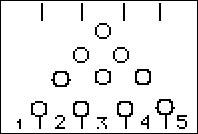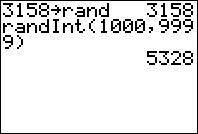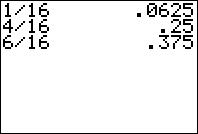# Activities

••• ##### Subject Area

• Math: Middle Grades Math: Statistics and Probability

• ##### Author6-8

45 Minutes

• ##### Device
• TI-73 Explorer™
• TI-Navigator™
• ##### Software

LearningCheck™ Creator

• ##### Report an Issue#### Activity Overview

Students simulate a popular game, find experimental probabilities, and then compare those probabilities to the corresponding theoretical probabilities.

#### Key Steps

•Students will explore experimental and theoretical probability by simulating the Plinko! game.

•Using the random number generator, students will simulate the disk falling into one of the five slots. They will then use this data to create a histogram and to calculate the experimental probability of each outcome of the simulation.

•Students will then look at the class set of data before calculating the theoretical probability of each outcome. Theoretical probabilities are then compared to the experimental probabilities determined earlier.